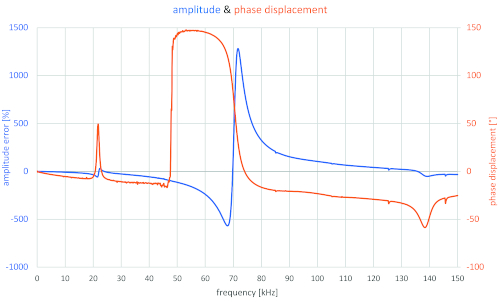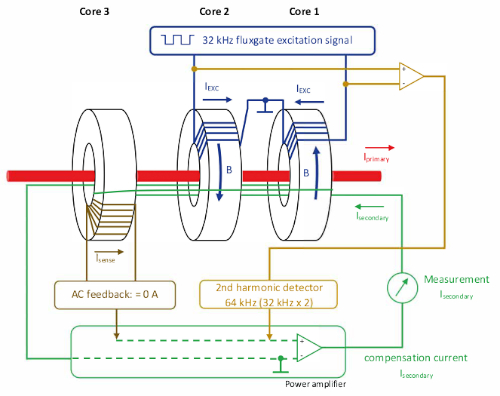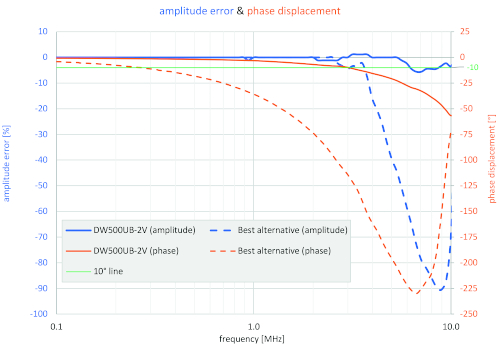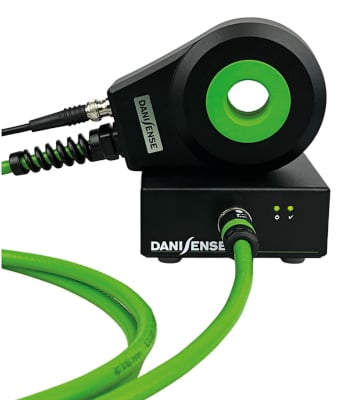New Industry Products

# Wide Bandwidth Current Transducers for Power Analysis

July 18, 2022 by Roland Buerger

## Power conversion products based on wide bandgap semiconductor technologies such as gallium nitride (GaN) and silicon carbide (SiC) are now operating at significantly higher switching frequencies which can generate almost perfect sinusoidal waveforms.

Filter elements such as chokes and capacitors can be made smaller and lighter because the inductance values of the chokes and the capacitances of the capacitors are inversely proportional to the switching frequency. In the future, SiC- and GaN-based components will continue to gain acceptance in more applications. This article explains a new approach and introduces a series of current transducers with the capability to handle the wide bandwidths now required.

### Power Analyzers

Power analyzers usually use the following basic formula for active power calculation.

$P_{avg}=\frac{1}{T} \int\limits_{0}^{T}v(t)\times i(t)\times dt$

Thus, the digitized instantaneous values of the voltage v(t) and the current i(t) are multiplied together and the results are summed up over a defined time window. Basically, DC components, all harmonic and non-harmonic components up to the bandwidth limit or filter cut-off frequency of the power analyzer are taken into account. Power analyzers in the premium segment already operate up to a frequency of 10 MHz. In most cases, the voltage signal is processed directly by the power analyzer so that the complete bandwidth of the power analyzer can be used.

For current measurements of greater than 30 A, galvanically isolated current sensors are often used, which must transmit the primary signal to the secondary side with high accuracy. These current sensors have a copper wire winding and an iron core as their main components. Also, Rogowski coils consist of a coil body wound with copper wire. This construction results in a winding inductance and also unwanted capacitances that are always formed between the individual windings and between the individual winding layers. Accordingly, each copper wire winding represents a potential oscillating circuit. With Thomson’s oscillation equation it is possible to calculate the resonant frequency.

$f_{r}=\frac{1}{2\times\pi\times\sqrt{LC}}$

Based on the formula, it can be stated that the larger the capacitance of the coil, the smaller the resonant frequency at constant inductance. The following figure shows a partially wound iron core. The complete secondary winding is divided into two coils and connected in series. Both secondary coils have several winding layers.

The individual capacitances are connected in parallel in the electrical equivalent circuit, which means that the total capacitances in one winding are summed together. Due to the coils being connected in series, the total capacitance of one coil has to be divided by two. The total capacitance of one coil, therefore, increases with the number of layers. Current sensors with more turns and more layers should, accordingly, have a lower resonant frequency.

This hypothesis has already been verified in practice by frequency measurements on inductive current transformers. In Figure 2, inductive current transformers of the same type with different turn ratios have been measured up to 35 kHz. The secondary current was always 1 A at primary rated current. As a result, the 4000 A model had more copper wire turns and more winding layers compared to the other devices.##### Figure 1. Partially wound current transformer with the electrical equivalent circuit for the secondary winding. Image used courtesy of Bodo’s Power Systems

The first resonance of the 4000 A current transformer is at approximately 9,570 Hz. The resonant frequency of the 3000 A device is approximately 13,000 Hz. The current characteristics show that the secondary signal is not only damped in the area of the resonance points but can also be amplified. Furthermore, a not insignificant phase shift also occurs.##### Figure 2. Frequency response of three inductive current transformers with different ratios. Image used courtesy of Bodo’s Power Systems

If the current sensor manufacturers define bandwidths, the power calculation should also be limited to this range. Otherwise, considerable inaccuracies are to be expected in the frequency range beyond this. This conclusion is further supported when considering the frequency response of a 5 A current clamp. The clamp is specified up to 20 kHz according to the data sheet. Directly after the 20 kHz, the first resonance point can be detected. A further resonance point can be detected between 60 and 80 kHz. In this range, amplitude values with an increase of approximately 1,270 percent can be displayed on the secondary side.

Generally, manufacturers are uncomfortable showing the sometimes chaotic-looking curves of their current sensors beyond the approved measuring range.##### Figure 3. Frequency response of a 5 A current clamp. Image used courtesy of Bodo’s Power Systems

However, the user must expect undesired curve characteristics in the non-defined higher frequency range of the sensors employed. A strong damping of the amplitudes above the specified frequency range is not common.

Also, the high-precision current sensors, which operate according to the flux-gate principle and have already been used for decades for power measurement, consist of several wound iron cores in combination with the necessary electronics.##### Figure 4. General principle of a balanced flux-gate transducer. Image used courtesy of Bodo’s Power Systems

Current components that are significantly above 10 kHz are transmitted passively via the third core. This means that above 10 kHz the sensor operates like a current transformer, according to the transformer principle.

The pioneers of flux-gate technology have now successfully optimized this third core for transmissions up to 10 MHz. The new DW500UB-2V current transducer from Danisense has a ratio of 500 A to 2 V and is currently the leading flux-gate transducer in the field with its frequency response up to 10 MHz.

If the current transducers, like the power analyzer, can now handle measurements up to 10 MHz, highly accurate and reliable power measurements should also be possible in the future when testing silicon carbide-based converters. The switching frequencies here are often 50 to 100 kHz. However, in addition to this switching frequency, multiples of the switching frequency are formed with corresponding sidebands. This phenomenon is described by the following formula.

f = n x fc ± 2 x n x f1

f = frequency of detectable amplitudes

n = multiplier (1;2;3;…)

fc = switching frequency of PWM

f1 = fundamental frequency of the grid

If a converter is now pulsed at 100 kHz, we can expect damped amplitudes also at 200, 300 and 400 kHz with the corresponding sidebands. In order to accurately measure the active power occurring in this situation, it must be noted that the phase displacement should also be as small as possible in addition to the amplitude error, since there is a significantly smaller power factor in the higher-frequency range. As a result, the influence of the phase displacement on the accuracy of the active power calculation increases significantly. The measurement equipment for the power analysis should therefore be able to cover the largest possible frequency range with regard to the amplitude error and the phase displacement in order to be able to exclude inaccuracies in the higher-frequency range.##### Figure 5. Frequency response of the new high bandwidth DW500UB-2V current transducer. Image used courtesy of Bodo’s Power Systems##### Figure 6. DW500UB-2V. Image used courtesy of Bodo’s Power Systems

Besides the power measurement, the high bandwidth is also useful to detect fast transients, inrush currents, surge currents and steepness of the current rise. The bigger phase shift (< -10 °) from 3 MHz onwards is less important here than for the power measurement.

The new DW500UB-2V has a voltage output signal of 2 V at 500 A. The full aluminum body ensures superior EMI shielding and extended operating temperature range.